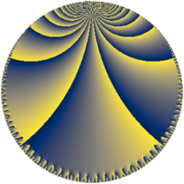# Properties

 Label 192.8.kLevel $192$ Weight $8$ Character orbit 192.k Rep. character $\chi_{192}(47,\cdot)$ Character field $\Q(\zeta_{4})$ Dimension $108$ Sturm bound $256$

# Related objects

## Defining parameters

 Level: $$N$$ $$=$$ $$192 = 2^{6} \cdot 3$$ Weight: $$k$$ $$=$$ $$8$$ Character orbit: $$[\chi]$$ $$=$$ 192.k (of order $$4$$ and degree $$2$$) Character conductor: $$\operatorname{cond}(\chi)$$ $$=$$ $$48$$ Character field: $$\Q(i)$$ Sturm bound: $$256$$

## Dimensions

The following table gives the dimensions of various subspaces of $$M_{8}(192, [\chi])$$.

Total New Old
Modular forms 464 116 348
Cusp forms 432 108 324
Eisenstein series 32 8 24

## Trace form

 $$108 q + 2 q^{3} + 8 q^{7} + O(q^{10})$$ $$108 q + 2 q^{3} + 8 q^{7} - 4 q^{13} + 60588 q^{19} - 4376 q^{21} - 238042 q^{27} - 4 q^{33} - 4 q^{37} + 283948 q^{39} - 752844 q^{43} - 156252 q^{45} + 9882508 q^{49} - 1121592 q^{51} - 4191000 q^{55} - 2279892 q^{61} - 1552540 q^{67} + 4372 q^{69} + 886066 q^{75} - 4 q^{81} + 7846496 q^{85} + 40789116 q^{87} + 1590680 q^{91} - 14495396 q^{93} - 8 q^{97} + 4701076 q^{99} + O(q^{100})$$

## Decomposition of $$S_{8}^{\mathrm{new}}(192, [\chi])$$ into newform subspaces

The newforms in this space have not yet been added to the LMFDB.

## Decomposition of $$S_{8}^{\mathrm{old}}(192, [\chi])$$ into lower level spaces

$$S_{8}^{\mathrm{old}}(192, [\chi]) \cong$$ $$S_{8}^{\mathrm{new}}(48, [\chi])$$$$^{\oplus 3}$$